Email Us: info@lupinepublishers.comCall Us: +1 (914) 407-6109   57 West 57th Street, 3rd floor, New York - NY 10019, USA

# Lupine Publishers Group

## Lupine Publishers

Submit Manuscript

ISSN: 2637-4676# About the Method of Calculating Pressure Redistribution in the Elastic Aquifer at Pumping Water Through Flowing Wells Volume 5 - Issue 2

NG Aloyan*

• National Agrarian University of Armenia

Received: October 27, 2018;   Published: October 30, 2018

Corresponding author: NG Aloyan, Department of Hydraulics, Armenia

DOI: 10.32474/CIACR.2018.05.000207

## Opinion

The article considers the problem of piezometric head redistribution when water intake is performed from the elastic aquifer through flowing wells. By using dimension theory and the method of least squares, we process the simulation results got from the simulation studies of flowing wells on the constant grid mathematical model and develop approximate formula for determining the pressure drawdown at any point of the suggested aquifer. Its checkup with the data of simulation and field studies has shown a satisfactory result.

Keywords: Flow rate; Flowing well; Elasticity; Piezometric head; Drawdown

## Introduction

The rate of flowing wells, as well as their head in them gradually decreases over time. Thus, for hydrological calculations there have been not accepted the Theis and Jacob’s formulas derived respectively for the wells that work at constant rate or with constant pressure in the well . The authors in the work  suggest the design formula for determining the pressure drawdown in the elastic aquifer at pumping water out through flowing wells. Though, this formula for engineering use is a kind of uncomfortable, thus, herein we suggest though approximate but comparatively simple kind of the design formula.

## Materials and Methods

The mentioned work  suggests graph dependency between dimensionless complexes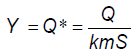and(Figure 1), at the processing of the results of simulation studies of flowing wells on the grid mathematical model of hydro integrator type [3,4]. Herein are marked:– well rate,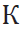– filtration coefficient of aquifer, m–its thickness, S–piezometric head drawdown at distancer from the well,piezo conductivity of the aquifer, µ* - elastic yield coefficient.

The function curve in Figure 1 has well-expressed hyperbolic shape. Let us proxy it by the formula:a and b are constant coefficients which we will define with the help of the method of least squares.

By taking the logarithm of the equation (1), we getAccording to the method of least squares, the coefficients a and b will have good value if the sum of squared deviations is minimal, i.e.where N – the number of calculated values of dimensionless complexes or number of dots put on the graph in Figure 1 Considering the terms of function extremum f ( a ,b), we will get the following equations: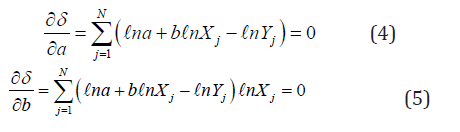After elementary transformations, these equations will get the form: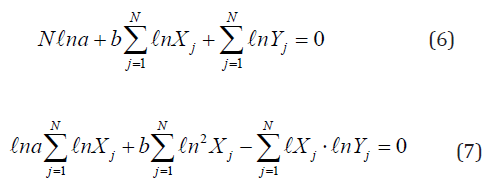To determine the coefficient a , from the equation (6) we will getFrom the equations (7) and (8), to determine the value of coefficient b, we will get the following dependency:The values of the calculated sums of the dimensionless complexes and their products, owned from the work [5-7], are as follows: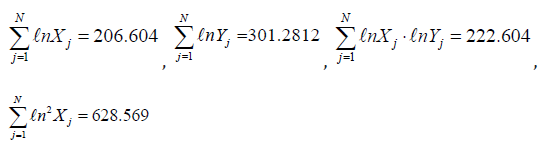where N= 151

By inserting these values in the equations (9) and (8), for the coefficients b and a we will get: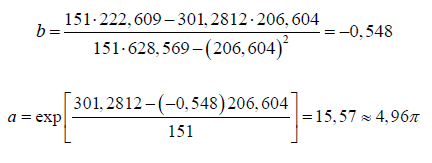Then the equation (1) will get the following form: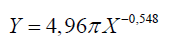## Conclusion

By replacing X and Y with the values of dimensionless complexes, after some transformations, to determine the pressure drawdown at any point of the aquifer, at any time, we will get the approximate formula in its final form:The formula checkup with the data of simulation and field studies in fact has shown a satisfactory result.

Close## Online Submission System

### Submit ManuscriptClick here for Manuscript Sample Templates### Drag and drop files here

or

( For multiple files submission, zip them in a single file to submit. For file zipping software Download )